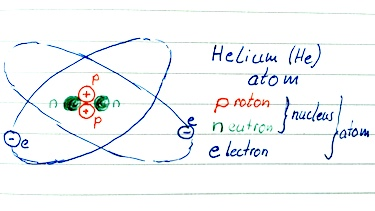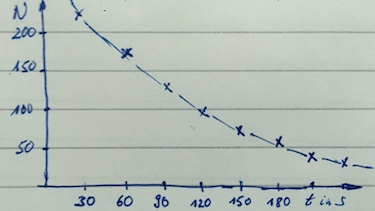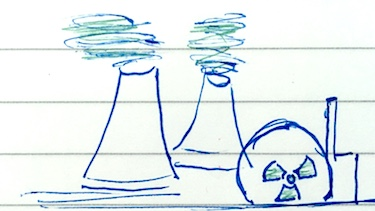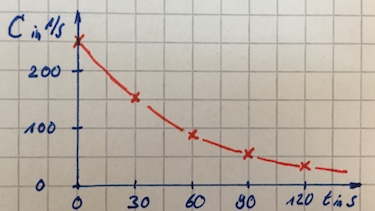Radioactivity is the emission of radiation from unstable nuclei. There are different types of radiation: $\alpha$, $\beta$ and $\gamma$-rays.

1 Structure of the atomTo understand radioactivity it is important to understand the structure of the atom, described by Niels Bohr. The nucleus consists of positively charged protons and neutrons. Negatively charged electrons orbit the nucleus in stationary states. The charge of an electron is $-e=1.6\cdot 10^{-19}\:$C, the charge of a proton is $+e$.

$$^4_2\text{He}$$

The element Helium He consists of two protons, two neutrons and two electrons. The nucleon number 4 indicates the number of protons and neutrons of the nuclide. The proton number or atomic number 2 indicates the number of protons of the nuclide and is specific for each element.Isotopes have the same proton number but differ in neutron number.

$$^3_2\text{He}$$

This isotope of Helium has two protons but only one neutron. The more neutrons a nuclide has, the heavier it is and is thus likely to be more unstable.

Radioactivity refers to the property of an unstable nucleus by emitting ionized rays and particles. The nucleus is changing into a different nucleus while emitting quantum like alpha-, beta- particles or gamma-rays. All these have destructive energy, even if it is not directly perceptible.

3 $\alpha$, $\beta$ and $\gamma$ radiationWhile the nucleus undergoes a decay often alpha-particles are emitted. They consist of helium nuclei without electrons. The atomic number will decrease by two and the nuclide number by four. They can already be absorbed by paper.

Beta-particles (electrons) are a result of a nucleon decaying into a proton. The atomic order will increase by one, while the nuclide number will remain equal. Beta particles can be absorbed by acrylic glass or metal layers.

Gamma rays result from atoms with excited state during decay. While turning back into non excited state the nuclei emit high energy Gamma-rays with a wavelength even shorter than X-rays. The atomic number will not change but only thick Pb-layers can absorb these electro-magnetic rays.Certain unstable nuclides do have different decay processes. The naturally existing Uranium-238 decays to Thorium-234. After 14 further decays it ends with the stable Lead-206.

$${^{238}_{92}\text{U} \xrightarrow[4.5\cdot 10^9 \text{a}]{\alpha}}\: {^{234}_{90}\text{Th} \xrightarrow[24.1 \text{d}]{\beta}}\: \: ... \: {^{206}_{82}\text{Pb}}$$

After a certain time, the half life, the amount of a nuclide is divided by two. For example U-238 has a half life of $4.5 \cdot 10^{9}\:$years while Th-234 has a half life of only 24 days. Every alpha particle or other radioactive ray has a certain energy in dependence of the nuclide which undergoes the decay.

5 Exponential decayThe radioactive decay can be described as follows: The amount $N$ of a nuclide after time $t$ can be calculated with the decay constant $\lambda$ and the incident amount of the nuclide $N_0$:

$$N(t)=N_0 \cdot e^{-\lambda t}$$

The half life can be derived by solving $N_0/2=N_0\cdot e^{-\lambda t_{1/2}}$:

$$t_{1/2}=-\frac{\ln(0.5)}{\lambda}$$
6 applicationsRadioactive nuclides appear in nature but also exist in nuclear reactors. Further applications are X-rays, the determination of the age of rocks - carbon dating and radio therapy.

Which statement is correct?

#### Question 2 Alpha-particle, Beta-particle, Gamma-rays

 property $\alpha$-particle $\beta$-particle $\gamma$-ray nature 2 pro­tons + 2 neu­trons an electron electro­magnetic wave masss $4\:$u $0.00055\:$u $0$ speed 5 % the speed of light 98 % the speed of light speed of light
1. Describe the charge of $\alpha$-particles, $\beta$-particles and $\gamma$-rays.
2. Describe how $\beta$-particles are generated.
3. Describe the existence of $\gamma$-rays.
4. ${^{238}_{92}\text{U}}$ undergoes decay by emitting an $\alpha$-particle and then a $\beta$-particle. State the change in mass of the nuclide.
5. Explain the meaning of isotopes.
1. charge of $\alpha$-particles: $2e$,
charge of $\beta$-particles: $-e$,
charge of $\gamma$-rays: $0$
2. Beta-particles (electrons) result by the decay of a nucleon into a proton.
3. Gamma rays result from atoms with excited state during decay by turning into non-excited state.
4. mass: $m_{Pb}=238\:\text{u}$, $m_{Th}=234\:\text{u}$, $m_{Pa}=234\:\text{u}$
5. Isotopes are atoms that have the same proton number, but different number of neutrons.

1. Draw the decay chain of ${^{238}_{92}\text{U}}$.
2. Justify why isotope with a short half time are less numerous.
3. Lead Pb has a proton number of 82. Write the nuclear equation for the emission of an $\alpha$-particle from polonium-214 ${^{214}_{84}\text{Po}}$.
4. Cobalt Co has a proton number of 27. Write the nuclear equation for the emission of an $\beta$-particle from iron-56 ${^{56}_{26}\text{Fe}}$.${^{238}_{92}\text{U} \xrightarrow[]{\alpha}}\: {^{234}_{90}\text{Th} \xrightarrow[]{\beta}}\: {^{234}_{91}\text{Pa} \xrightarrow[]{\beta}}\:$ ${^{234}_{92}\text{U} \xrightarrow[]{\alpha}}\: {^{230}_{90}\text{Th} \xrightarrow[]{\alpha}}\: {^{226}_{88}\text{Ra} \xrightarrow[]{\alpha}}\:$ ${^{222}_{86}\text{Rn} \xrightarrow[]{\alpha}}\: {^{218}_{84}\text{Pa} \xrightarrow[]{\alpha}}\: {^{214}_{82}\text{Pb} \xrightarrow[]{\beta}}\:$ ${^{214}_{83}\text{Bi} \xrightarrow[]{\beta}}\: {^{214}_{84}\text{Po} \xrightarrow[]{\alpha}}\: {^{210}_{82}\text{Pb} \xrightarrow[]{\beta}}\:$ ${^{210}_{83}\text{Bi} \xrightarrow[]{\beta}}\: {^{210}_{84}\text{Po} \xrightarrow[]{\alpha}}\: {^{206}_{82}\text{Pb}}$
2. Isotope with a short half time are less numerous since they decay faster then their previous isotope.
3. decay of polonium-214: ${^{214}_{84}\text{Po} \xrightarrow[]{\alpha}}\: {^{210}_{82}\text{Pb}}$
4. decay of iron-56: ${^{56}_{26}\text{Fe} \xrightarrow[]{\beta}}\: {^{56}_{27}\text{Co}}$

### Relax ...

Flat earth theory.

#### Question 4 Exponential decay

The following count rate from a radioactive source was measured.

 $t$ in s 0 30 60 90 120 count rate in 1/s 160 110 71 47 32
1. Plot a graph of the count rate versus time.
2. Use the graph to determine $\lambda$.
3. Determine the half life $t_{1/2}$.
4. Calculate the time for $N(t)=N_0/4$.
1. count rate versus time:2. Determine the decay constant $\lambda$ from graph:Determine the decay constant $\lambda$ by calculation:
$152=250\cdot e^{-\lambda \cdot 30\:\text{s}} \Leftrightarrow \lambda = 1/60\:1/\text{s}$
3. $t_{1/2}=-60\cdot ln(1/2)\:\text{s}$
4. $t_{1/4}=-60\cdot ln(1/4)\:\text{s}$

### Terms and phrases

 atomic nucleus The central area of an atom, consisting of protons and neutrons. nuclide An atom with specific number of protons and neutrons. radioactivity Radioactivity refers to the property of an unstable nucleus by emitting ionized rays and particles. radio­active decay The nucleus is changing into a different nucleus while emitting quantum like alpha-, beta- particles or gamma-rays. count rate$C$ The number of measured radioactive particles per second.
 exponential decay If a radioactive material undergoes decay the number of nuclei $N$ decreases by an exponential function $N(t)=N_0\cdot e^{-\lambda t}$. $N_0$ the initial number of nuclei, $\lambda$ the decay constant. The count rate $C$ or the activity $A$ also follow this law. decay constant $\lambda$ in 1/s $1/\lambda$ is the time where the number of nuclei is reduced by 37 %. It also refers to the initial gradient of the decay-graph. half life $t_{1/2}$ The half life $t_{1/2}$ refers to the time where the numbers of nuclei are divided by two. applications of radioactivity nuclear power plants, X-rays, determine the age of rocks - carbon dating, radio therapy